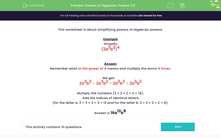# Powers of Algebraic Powers (3)

In this worksheet, students must simplify powers of algebraic powers.Key stage:  KS 3

Curriculum topic:   Algebra

Curriculum subtopic:   Simplify Algebraic Expressions to Maintain Equivalence

Difficulty level:#### Worksheet Overview

This worksheet is about simplifying powers of algebraic powers.

Example

Simplify:

(2a3b2)4

Remember what to the power of 4 means and multiply the terms 4 times.

We get:

2a3b2 × 2a3b2 × 2a3b2 × 2a3b2

Multiply the numbers (2 × 2 × 2 × 2 = 16).

Add the indices of identical letters

(for the letter a: 3 + 3 + 3 + 3 = 12 and for the letter b: 2 + 2 + 2 + 2 = 8).